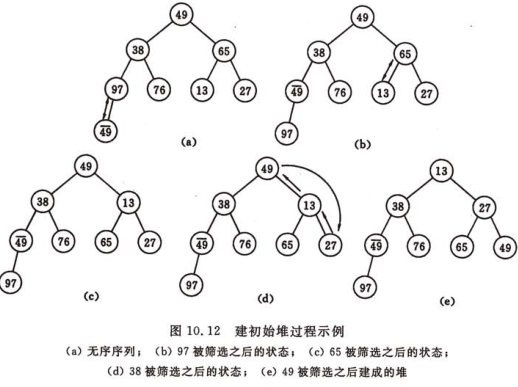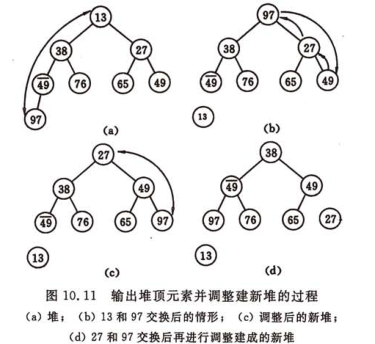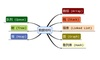• *堆排序（heapsort） 是选择排序的升级 降低了排序函数的冗余性 *堆排序分为 大顶堆 和小顶堆 大顶堆为堆顶为最大元素 小顶堆为堆顶为最小元素 *先建立堆 再调整 最后输出 堆的元素 *建立在二叉树的基础上 */ void...
/*
*堆排序（heapsort）   是选择排序的升级版  降低了排序函数的冗余性
*堆排序分为 大顶堆 和小顶堆   大顶堆为堆顶为最大元素   小顶堆为堆顶为最小元素
*先建立堆 再调整 最后输出  堆的元素
*建立在二叉树的基础上
*/
void HeapSort(int *s,int length);//堆排序函数
void HeapAdjust(int *s,int i,int length);//堆排序的辅助函数
void main()
{
int m;
int n;//待排序的数组
int i;
printf("请输入要输入的数据个数:\n");
scanf("%d",&m);
printf("请依次输入数据:\n");
for(i=0;i<m;i++)
scanf("%d",&n[i]);
printf("堆排序遍历结果为:\n");
HeapSort(n,m);
for(i=0;i<m;i++)
printf("%d\t",n[i]);

}
void HeapSort(int *s,int length)
{
int i;
int temp;//交换中间变量
//创建大顶堆
for(i=length/2-1;i>=0;--i)//length/2-1为最后 一个非叶子节点
HeapAdjust(s,i,length);
//将堆中的元素按递增的排序输出
//将最后一个元素和第一个元素替换 始终保持每次循环调整之后最后一个元素是最大元素
//循环条件是为了让每次调整之后的第一个元素为最大元素  以便之后的数值交换
//直到循环到第一个元素结束  到main函数中进行输出
for(i=length-1;i>0;--i)//将最后一个元素和第一个元素进行交换
{
temp=s[i];//第一个元素的值和最后一个元素的值进行交换
s[i]=s;
s=temp;
HeapAdjust(s,0,i);//用于调整第一个元素的值  使其保持为调整后的最大值
// 为下一次循环创造条件
}
}
void HeapAdjust(int *s,int i,int length)
{
int child;//孩子节点在数组中下标
int temp;//中间变量
for( ;2*i+1<length;i=child)
{
child=2*i+1;//得到根节点的左孩子节点在数组中的下标
if(child<length-1&&s[child]<s[child+1])//左节点小于其右节点的值
//并判断是否存在其右节点
child++;//将孩子节点的下标指向其右孩子节点
if(s[i]<s[child])
{
temp=s[i];//进行数值交换
s[i]=s[child];
s[child]=temp;
}
else
break;

}
}




展开全文• 文章目录堆排序 堆排序
文章目录堆排序
堆排序
堆排序的由来还得说到简单选择排序，由简单选择排序中的在进行剩余的n -2个数据比较时若能利用前n-1次比较的所得信息，则可以减少以后各趟排序的比较次数，由此联想出锦标赛排序也就是树形排序，但是树形排序的辅助存储空间较多，和“最大值”进行比较多余的比较等缺点，因此，在1964年威洛姆斯提出了堆排序，堆排序灵活的应用了最堆的特性来达到选择的目的。

堆排序只需要一个记录大小的辅助空间，每个待排序的记录仅占有一个存储空间。

要进行堆排序首先我们要先建一个最大（小）堆，（这里关于如何建最堆以下代码中有体现，）

图示：然后是在输出堆顶元素后再次调整剩余元素为一个新的最堆这个过程也叫作“筛选”。

图示：代码：
Sort.h

#pragma once

#include <stdio.h>
#include <stdlib.h>
#include <assert.h>

#define N 10

void InputData(int* d);//输入数据
void PrintData(int* d);//输出数据

void InsertSort(int* d);//直接插入排序
void ShellSort(int* d, int length);//希尔排序
void SelectSort(int* d, int len);//选择排序

typedef int HPDataType;
typedef struct Heap
{
HPDataType* a;
int num;
int capacity;
}Heap, *pHeap;//创建堆
void HeapSort(HPDataType* d, int n);//堆排序
pHeap HeapCreat(HPDataType* d, int n);//创建大堆
void HeapDestory(pHeap hp);//销毁堆
void HeapPrint(Heap hp);//堆打印
void AdjustDown(HPDataType* a, int n, int root);//堆内渗透函数

Sort.c
#include "Sort.h"

void InputData(int* d)
{
int i = 0;
printf("输入数据：\n");
for (i = 0; i < N; i++)
{
printf("	 d[%d]:", i);
scanf("%d", &d[i]);
printf(" ");
}
printf("\n\n");
}

void PrintData(int* d)
{
int i = 0;
printf("输出数据：");
for (i = 0; i < N; i++)
{
printf("%d ", d[i]);
}
printf("\n\n");
}

void Swap(int* d1, int *d2)
{
assert(d1 && d2);
int tmp = 0;
tmp = *d1;
*d1 = *d2;
*d2 = tmp;
}

//直接插入排序
//假设待排序的记录存放在数组d[0..N-1]中。
//初始时，d自成1个有序区，无序区为R[1.N-1]。
//从i=1起直至i=N-1为止，依次将d[i]插入当前的有序区d[1..N-1]中，
//生成含N个记录的有序区。

void InsertSort(int * d)
{
assert(d);

int i = 0;
int j = 0;
int right = 0;
for (i = 1; i < N; i++)
{
//在原来的数组里操作，比较一次移动一次
right = i;//记录要载入数据的位置，哨兵
for (j = right - 1; j >= 0; j--)
{
if (d[right] < d[j])
{
Swap(&d[right], &d[j]);
}
right--;
}
}
printf("排序成功!\n\n");
}//InsertSort

//基础：插入排序在对几乎已经排好序的数据操作时，效率高，即可以达到线性排序的效率。
//通过分组使数组渐变有序
//最后在直接插入排序
void ShellSort(int *d, int length)//希尔排序
{
assert(d);

int i = 0, j = 0;
int gap = 0;//步长即分组的个数
int temp = 0;//哨兵

for (gap = length / 2; gap > 0; gap /= 2)//完成分组
{
for (i = gap; i < length; i++)//寻找分组后的元素
{
temp = d[i];//哨兵位
for (j = i - gap; j >= 0 && temp < d[j]; j -= gap)//根据下一个分组的下标寻找上个分组的元素
{
d[j + gap] = d[j];
}
d[j + gap] = temp;//填补上一个分组的相应位置元素
}
}
printf("排序成功!\n\n");
}//ShellSort()

int SelectMinKey(int* d, int i, int len)
{
assert(d);
int min = i;

for (int cur = i + 1; cur < len; ++cur)
{
if (d[min] > d[cur])
{
min = cur;
}
}
return min;
}

void SelectSort(int* d, int len)//选择排序
{
assert(d);

for (int i = 0; i < len; ++i)//选择第i小的元素
{
int j = SelectMinKey(d, i, len);
if (i != j)
{
Swap(&d[i], &d[j]);//找到最小值并与i位只交换
}
}
printf("排序成功!\n\n");

}//SelectSort();

void HeapSort(HPDataType* d, int n)//堆排序
{
assert(d);
int i = 0;
Heap hp;

//通过堆的属性来寻找最大（小）值，升序：大堆， 降序：小堆
//创建大堆
hp.a = (HPDataType*)malloc(sizeof(HPDataType)* n);//堆元素开辟空间
assert(hp.a);//防止开辟失败
hp.capacity = n;
hp.num = n - 1;//元素下标0 - (n-1)
for (i = 0; i < n; i++)
{
hp.a[i] = d[i];
}
//向下调整为大堆
for (i = ((n - 2) / 2); i >= 0; --i)
{
AdjustDown(hp.a, hp.num + 1, i);
}
printf("大堆：");
for (i = 0; i < hp.num; i++)
{
printf("%d ", hp.a[i]);
}
printf("\n\n");

//排序
int end = hp.num;
while (end >= 0)
{
//交换root和最后一个节点的数据
Swap(&(hp.a), &(hp.a[end]));
--end;//下标前进1
n--;//数据减一
AdjustDown(hp.a, n, 0);
}

printf("排序后：");
for (i = 0; i <= hp.num; i++)
{
printf("%d ", hp.a[i]);
}
printf("\n\n");
}

void AdjustDown(HPDataType* a, int n, int root)//堆内渗透函数
{

int parent = root;
int child = parent * 2 + 1;
while (child < n)
{
//选左右中大的一个
if (a[child + 1] > a[child] && child + 1 < n)
{
child += 1;
}
if (a[child] > a[parent])
{
Swap(&a[child], &a[parent]);//在函数外部进行交换要传址
parent = child;
child = child * 2 + 1;
}
else
{
break;
}
}
}

main.c
#include "Sort.h"

void Test()
{
int d[N] ={15, 45, 23, 78, 63, 5, 23, 58, 31, 10};

/*int d[N];
InputData(d);*/
PrintData(d);

/*InsertSort(d);
PrintData(d);*/

/*ShellSort(d, sizeof(d)/sizeof(d));
PrintData(d);*/

/*SelectSort(d, sizeof(d) / sizeof(d));
PrintData(d);*/

HeapSort(d, sizeof(d) / sizeof(d));

}

int main()
{
Test();
system("pause");
return 0;
}



展开全文• 之前写了直接插入和折半插入的排序算法，这次的是堆排序算法，相比前两种，这种的时间复杂度更低一些，在一些题目里面，有可能你用其他的排序方法就会超时，而用堆排序就不会。 堆的定义什么的就略去了 代码： #...
之前写了直接插入和折半插入的排序算法，这次的是堆排序算法，相比前两种，这种的时间复杂度更低一些，在一些题目里面，有可能你用其他的排序方法就会超时，而用堆排序就不会。

堆的定义什么的就略去了

代码：

#include <stdio.h>
#include <stdlib.h>
#include <malloc.h>
#define MAX_SIZE 10000
//typedef struct {
//    int r[MAX_SIZE+1];
//    int Length;
//}
void HeapAdjust(int *a,int head,int tail);
void heapSort(int a[],int len);

int main(){
int M,N;
scanf("%d%d",&M,&N);
int a[M];
int i;
for(i = 0; i < M;i++){
scanf("%d",&a[i]);
}
int n = M;
heapSort(a,n);

for(i = M-1; i >= M-N && i>= 0; i--){
if(i == M-1)
printf("%d",a[i]);
else
printf(" %d",a[i]);
}
}

void HeapAdjust(int *a,int head,int tail){
int tmp = a[head];
int j = 2*head+1;
int i = head;
while(j <= tail){
if(j < tail && a[j] < a[j+1])
++j;
if(tmp < a[j]){
a[i] = a[j];
i = j;
j = 2*i+1;
}
else
break;
}
a[i] = tmp;
}
void heapSort(int a[],int n){
int i;
int tmp;
for(i = n/2-1; i >= 0; --i){
HeapAdjust(a,i,n-1);
}
for(i = n-1; i > 0;--i){
tmp = a;
a = a[i];
a[i] = tmp;
HeapAdjust(a,0,i-1);
}
}


注：可以去B站搜一下堆排序，有个相关视频可以帮助理解！
展开全文• 算法：把未排序数据一个一个放入里，然后再一个一个的取出来。我们今天使用上一个博客写的大顶堆MaxHeap.h//MaxHeap.h #ifndef _MAX_HEAP_ #define _MAX_HEAP_template class MaxHeap{ public: MaxHeap(int mx ...
算法：把未排序的数据一个一个放入堆里，然后再一个一个的取出来。

我们今天使用上一个博客写的大顶堆MaxHeap.h

//MaxHeap.h
#ifndef _MAX_HEAP_
#define _MAX_HEAP_

template<class T>
class MaxHeap{
public:
MaxHeap(int mx = 10);
virtual ~MaxHeap();
bool IsEmpty();
void Push(const T&);
void Pop();
const T& Top() const;
private:
T* heapArray;
int maxSize;
int currentSize;

void trickleUp(int index);
void trickleDown(int index);
};

template<class T>
MaxHeap<T>::MaxHeap(int mx = 10){
if (mx < 1) throw "max size must be >= 1";
maxSize = mx;
currentSize = 0;
heapArray = new T[maxSize];
}

template<class T>
MaxHeap<T>::~MaxHeap(){
delete[]heapArray;
}

template<class T>
bool MaxHeap<T>::IsEmpty(){
return currentSize == 0;
}
template <class T>
void MaxHeap<T>::Push(const T&e){
if (currentSize == maxSize) throw "MaxHeap id full.";
heapArray[currentSize] = e;
trickleUp(currentSize);
currentSize++;
}

template<class T>
void MaxHeap<T>::trickleUp(int index){
int parent = (index - 1) / 2;
T bottom = heapArray[index];
while (index>0 && heapArray[parent] < bottom){
heapArray[index] = heapArray[parent];
index = parent;
parent = (parent - 1) / 2;
}
heapArray[index] = bottom;
}

template<class T>
const T& MaxHeap<T>::Top()const{
return heapArray;
}

template<class T>
void MaxHeap<T>::Pop(){
heapArray = heapArray[--currentSize];
trickleDown(0);
}

template<class T>
void MaxHeap<T>::trickleDown(int index){
int largerChild;
T top = heapArray[index];
while (index < currentSize / 2){
int leftChild = 2 * index + 1;
int rightChild = leftChild + 1;
if (rightChild < currentSize && heapArray[leftChild] < heapArray[rightChild])
largerChild = rightChild;
else
largerChild = leftChild;

if (top >= heapArray[largerChild])
break;
heapArray[index] = heapArray[largerChild];
index = largerChild;
}
heapArray[index] = top;

}
#endif

下面是我堆排序的主程序main.cpp

//main.cpp
#include<iostream>
#include"MaxHeap.h"

using namespace std;

int main(){
cout << "测试堆：" << endl;
MaxHeap<int> h(100);
int arr[] = { 62, 3, 90, 27, 33, 8, 12, 9, 43, 66 };
for (int i = 0; i < 10; i++)
h.Push(arr[i]);
for (int i = 0; i < 10; i++){
arr[i] = h.Top();
h.Pop();
}

for (int i = 0; i < 10; i++)
cout << arr[i] << " ";
cout << endl;
system("pause");
return 0;
}

总结：堆排序比较简单就是把数据一个一个push进堆，再一个一个从堆里取出来。
展开全文• 01-001数据结构的概念和基本术语、抽象数据类型的表示与实现 01-002算法设计的要求、算法效率的度量 02-001线性表的类型定义 02-002线性表的顺序表示与实现、线性表的基本操作 02-003单链表的创建与操作、加工型...
• //排序: /* 1、录入学生基本信息 ... 6、堆排序 7、2-路归并排序 8、输出学生信息 */ #include<stdio.h> #include<stdlib.h> typedef struct{ char name; int num; int score; ...
• 堆排序    堆排序属于选择排序类别，其算法步骤是：1、从无序序列建立最大堆（升序就构造最大堆）或者最小堆（降序就构造最小堆）2、利用构建的堆进行排序。    堆排序的平均时间复杂度和最坏时间复杂度均为在...Python
• /* *简单插入排序（以temp元素为中间值 控制循环条件 不满足条件进行交换） *插入排序（插入一个数据保持有序排列...*堆排序算法（利用二叉树点的储存规则 保证树中元素的顺序保持递增或者递减的规律） *基数排序算...
• 堆排序(Heapsort)是指利用堆积树（堆）这种数据结构所设计的一种排序算法，它是选择排序的一种。它是 通过堆来进行选择数据。需要注意的是排升序要建大堆，排降序建小堆。 2.图片解释算法 1.将第一个元素和最后一个...
• 高级排序算法：归并排序，堆排序，快速排序 插入排序就是在已经排序的数据中从大往小比较，出现比该数小的就插入到该位置后面。#include using namespace std;void InsertionSort(int *a, int n); int main(){ int...算法
• 完全二叉树叫做堆 完全二叉树就是在最后一个节点之前不允许有不满的节点（有空洞）用数组来做完全...删除根节点，先把根节点删除，然后把最后一个节点加到根上，然后和子代交换堆用来做优先队列，用来排序（堆排序完全二叉树
• 数据结构与算法实验报告 需求分析 问题描述在教科书中各种内部排序算法的时间复杂度分析结果只给出了算法执行时间的阶或大概执行时间试通过随机数据比较各算法的关键字比较次数和关键字移动次数以取得直观感受 基本...
• 10.4.3 堆排序 10.5 归并排序 10.6 基数排序 10.6.1 多关键字的排序 10.6.2 链式基数排序 10.7 各种内部排序方法的比较讨论 第11章 外部排序 11.1 外存信息的存取 11.2 外部排序的方法 11.3 多路平衡归并...
• C语言编写的数据结构演示...主要使用Dos演示数据结构的线性结构（数组，链表，串，队列和栈）、树（树的遍历，平衡二叉排序树）和排序结构（简单排序，堆排序，快速排序，归并排序），并且附有完整的程序设计报告。
• 10.4 选择排序 一简单选择排序 三堆排序 二树型选择排序 一简单选择排序 假设排序过程中待排记录序列的状态为 有序序列R[1.i-1] 无序序列 R[i.n] 第 i 趟 简单选择排序 从中选出 关键字最小的记录 有序序列R[1.i] ...
• 1.从未排序的序列中依次取出元素与已排序序列中的元素进行比较，将其放入已排序序列的正确位置上的方法（插入排序） ...析：讲的应该是冒泡排序的改进（即用flag标志是否已为顺序，若未发生交换则已排好序）算法
• 数据结构(C语言版) 非常不错的资源 第1章 绪论 1.1 什么是数据结构 1.2 基本概念和术语 1.3 抽象数据类型的表示与实现 1.4 算法和算法分析 1.4.1 算法 1.4.2 算法设计的要求 1.4.3 算法效率的度量 1.4.4 算法的...
• 数据结构与算法（C语言版数据结构和算法讲解（数组，链表，队列，排序，树，递归，，图................）
• 7.6 堆排序 7.7 多关键字排序 7.8 链表排序和索引表排序 7.9 内部排序小结 7.10 外部排序 7.11 参考文献和选读材料 第8章 Hash法 8.1 引言 8.2 静态Hash法 8.3 动态Hash法 8.4 Bloom滤波器 8.5 参考文献...
• 排序排序排序算法的评估指标排序算法的分类插入排序算法效率分析优化——折半插入排序希尔排序（Shell Sort）算法性能分析冒泡排序算法性能分析快速排序算法效率分析简单选择排序算法性能分析堆排序堆的定义建立大根...排序算法 快速排序 合并排序
• Williams在1964年发表的堆排序（heap sort），当时他提出了二叉堆树作为此算法的数据结构。 性质： 任意节点小于（或大于）它的所有后裔，最小元（或最大元）在堆的根上（堆序性）。 堆总是一棵完全树。即除了最...
• 数据结构自测题 (C 语言 ) 一单项选择 (每题 1分共 10 分) ( 答案及点评 ) 若广义表K满足head(K)=tail(K,贝U K为( ) ( ) B( ( ) ) C. ( ) ( ) ) D( ) ) ( 答案及点评 ) 若要求尽可能快地对实数数组进行稳定的排序...
• 数据结构》（c语言版）是为“数据结构”课程编写的教材，也可作为学习数据结构及其算法的C程序设计的参数教材。学了数据结构后,许多以前写起来很繁杂的代码现在写起来很清晰明了. 本书的前半部分从抽象数据类型的...
• 1.1 什么是数据结构 1.2 基本概念和术语 1.3 抽象数据类型的表现与实现 1.4 算法和算法分析 第2章 线性表 2.1 线性表的类型定义 2.2 线性表的顺序表示和实现 2.3 线性表的链式表示和实现 2.4 一元多项式的表示及相加...
• 数据结构》（C语言版）是为“数据结构”课程编写的教材，也可作为学习数据结构及其算法的C程序设计的参数教材。学了数据结构后,许多以前写起来很繁杂的代码现在写起来很清晰明了. 本书的前半部分从抽象数据类型的...
• 今天在《算法导论》第二看完了“堆排序”算法，就顺便用C语言实现了一下。  堆排序算法的核心思想，使用一种二叉堆的数据结构来存储数据，其中二叉堆（最小二叉堆）的主要性质为:  1 父节点小于所有的子节点......

# c语言版数据结构堆排序c语言 订阅数据结构 订阅